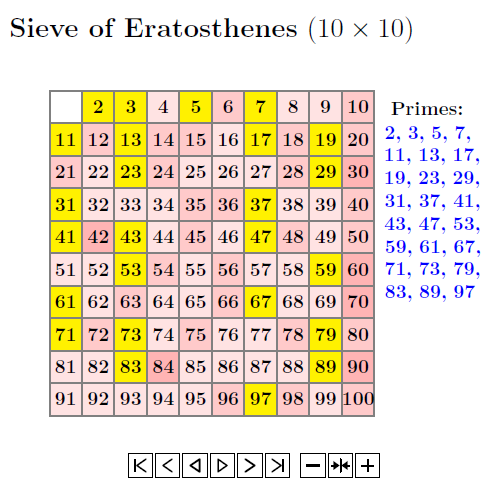$$\newcommand{\id}{\mathrm{id}}$$ $$\newcommand{\Span}{\mathrm{span}}$$ $$\newcommand{\kernel}{\mathrm{null}\,}$$ $$\newcommand{\range}{\mathrm{range}\,}$$ $$\newcommand{\RealPart}{\mathrm{Re}}$$ $$\newcommand{\ImaginaryPart}{\mathrm{Im}}$$ $$\newcommand{\Argument}{\mathrm{Arg}}$$ $$\newcommand{\norm}{\| #1 \|}$$ $$\newcommand{\inner}{\langle #1, #2 \rangle}$$ $$\newcommand{\Span}{\mathrm{span}}$$

6.1: Prime numbers

•• Contributed by Pamini Thangarajah
• Associate Professor (Mathematics & Computing) at Mount Royal University

$$\newcommand{\vecs}{\overset { \rightharpoonup} {\mathbf{#1}} }$$

$$\newcommand{\vecd}{\overset{-\!-\!\rightharpoonup}{\vphantom{a}\smash {#1}}}$$

Definition

Prime Numbers - integers greater than 1 with exactly 2 divisors: 1 and itself.
Let n be a positive integer greater than 1. Then n is called prime number if n has exactly two positive divisors, 1 and n.

Composite Numbers - integers greater than 1 which are not prime.

Note that: $$1$$ is neither prime nor composite.

Theorem 1:

Let $$n$$ be a composite number with exactly $$3$$ positive divisors. Then there exists a prime ($$p$$) such that n=p

Proof:

Let $$n$$ be a positive integer with exactly 3 positive divisors. Then two of the positive divisors are 1 and n.
Let $$d$$ be the 3rd positive divisor. Then $$1 < d < n$$ and the only positive divisors are $$1$$ and $$n.$$
Therefore $$d$$ is prime.
Since $$d \mid n$$, $$n=md, m \in\mathbb{Z_+}$$.
Since $$m \mid n$$ and $$1<m<n, m=d$$, n=d2.
Thus, $$d$$ is prime. $$\Box$$

Theorem 2:

Every composite number n has a prime divisor less than or equal to the $$\sqrt{n}$$. If p is a prime number and $$p \mid n$$, then $$1<p \leq √n.$$

Note

There are infinitely many primes, proved by Euclid in 100BC.

Example $$\PageIndex{1}$$: Method of Sieve of Eratosthenes

Examples of prime numbers are 2 (this is the only even prime number), 3, 5, 7, 9, 11, 13, 17, $$\ldots$$

Method of Sieve of Eratosthenes:Example $$\PageIndex{2}$$:

Is 225 a prime number?

Solution:

The prime numbers < √225 are as follows: 2, 3, 5, 7, 11, and 13. Since 172 is >225.

Since 223 is not divisible by any of the prime numbers identified above, 223 is a prime number.

Exercise $$\PageIndex{1}$$

Is 2011 a prime number?.

yes.

Prime divisibility Theorem

Let $$p$$ be a prime number and let $$a, b$$ be integers.

If $$p\mid ab$$ then $$p\mid a$$ or $$p\mid b$$.

Theorem

There are infinitely many primes.

Fundamental Theorem of Arithmetic (FTA)

Let $$n \in\mathbb{Z_+}$$, then n can be expressed as a product of primes in a unique way. This means n= P1n1,P2n2, ...., Pk nk , where $$P_1 <P_2< ... <P_k$$ are primes.

Note: Conjectures

Gildbach's Conjecture(1742)

Every even integer $$n >2$$ is the sum of two primes.

Goldbach's Ternary Conjecture:

Every odd integer $$n \geq 5$$ is the sum of at most three primes.